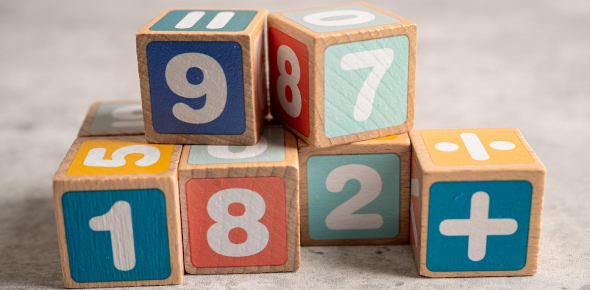# Quiz: Number System Class 9 MCQ Online Test

10 Questions | Attempts: 11991
ShareSettingsGet ready for this number system class 9 MCQ online test that we have made for you. This number system quiz is going to test as well as give you even more clarity and understanding. If you are a class 9 student or above, you should be able to pass this quiz with a very good score. So, will you be able to get a score of 80 on this quiz? Let us see! Best of luck to you!

• 1.
The value of 1.999... in the Vulgar Fraction is:
• A.

19/10

• B.

199999/100000

• C.

2

• D.

1/9

• 2.
Every Whole Number is not a:
• A.

Real Number

• B.

Natural Number

• C.

Integer

• D.

Rational Number

• 3.
The decimal expansion of 1.01001000100001000001..... is?
• A.

Non-Terminating

• B.

Non Repeating

• C.

Non Terminating but Repeating

• D.

Non-Terminating Non Repeating

• 4.
The square of an irrational number is always rational? Is the state true or false?
• A.

True

• B.

False

• 5.
The number of Rational Numbers between 15 and 18 is finite.
• A.

True

• B.

False

• 6.
Under-root 225 is Rational.
• A.

True

• B.

False

• 7.
Rational Number can never have Non-Terminating Non-Repeating Decimal Expansion.
• A.

True

• B.

False

• 8.
Under-root 0.4 is Rational.
• A.

True

• B.

False

• 9.
The Maximum Number of digits in the Repeating block of digits in the decimal expansion of 3/13 is [Blank]?
• 10.
The decimal representation of a Rational Number cannot be:
• A.

Terminating

• B.

Non- Terminating

• C.

Non Terminating but Repeating

• D.

Non-Terminating Non Repeating

## Related TopicsBack to top
×

Wait!
Here's an interesting quiz for you.